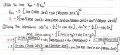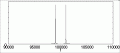# How to find the magnitude component at a given frequency with the input-output relationship and input

#### Minchuu

Joined May 13, 2021
17
Hello!

We were tasked to find the 100kHz magnitude component of a non linear device with a function $$V_{out}=5V_{in}^{2}$$ where $$V_{in}=cos(1000\cdot 2\pi\cdot t)+sin(1000002\pi\cdot t)$$.

So far I have,And now I have no idea where will I find the 100kHz component. Do I turn the frequency (Hz) to period (s) so I can insert it into t?

Any hints will be greatly appreciated, thank you very much!

#### Minchuu

Joined May 13, 2021
17
Someone pointed something out to me and I can't believe I didn't saw it before. The answer is already in my solution, lol. There is no 100kHz component because there is no 100k inside the cos nor sin. I thought I was just making mistakes in the equation. No need to reply to this thread further, thanks!

•ericgibbs

#### Papabravo

Joined Feb 24, 2006
17,240
I do not think that is correct. You may have arrived at what you think is the correct answer but for the wrong reason. Any time you have a nonlinear process, like multiplication, the result will have the original two frequencies and the sum and the difference. Since 100kHz is one of the original frequencies it will show up with a distinct magnitude in the result. The frequency components will be:
1. 1000 Hz. -- an original frequency
2. 100,000 Hz. -- an original frequency
3. !01,000 Hz. -- the sum of the two original frequencies
4. 99,000 Hz. -- the difference of the two original frequencies.
So yes you made a mistake in your equations, and there are no components at 2000 or 200,000 Hz.

•Deleted member 115935

#### neonstrobe

Joined May 15, 2009
178
...unless it is squared? (Hint: that's why we use RMS for AC power calculations).
the OP is right. There are 2kHz,200kHz, 99kHz and 101kHz components in the output.

#### Papabravo

Joined Feb 24, 2006
17,240
...unless it is squared? (Hint: that's why we use RMS for AC power calculations).
the OP is right. There are 2kHz,200kHz, 99kHz and 101kHz components in the output.
Odd. I've never seen that result before. It seems like the multiplication rule was a general result, independent of the two operands being identical. I think that is true for operands with a single frequency component. Here we have two operands, each with two frequency components, multiplied together. When you do that the sum of the product of two identical sine waves it will be twice the frequency and the difference will be zero. You also get the sum and the difference of the original frequencies. I'm less clear about why the original frequencies are suppressed, but I trust the algebra.

As a modestly interesting side note, doing a simulation almost always helps to clarify a concept. LTspice has a Control Library that is eminently suitable for this purpose. It confirms that the frequencies of the original signals are suppressed by the squaring operation.

Last edited:

#### neonstrobe

Joined May 15, 2009
178
You were probably thinking of a system which is basically linear with a non-linear component. That would give the original frequencies plus distortion/intermodulation components. But there would have been 2kHz and 200kHz if the non-linear part were square law.
You're welcome.

#### Papabravo

Joined Feb 24, 2006
17,240
You were probably thinking of a system which is basically linear with a non-linear component. That would give the original frequencies plus distortion/intermodulation components. But there would have been 2kHz and 200kHz if the non-linear part were square law.
You're welcome.
I was thinking the typical SSB transceiver where an RF carrier is modulated by an audio signal. You get USB and LSB as well as the two original frequencies from what is essentially a non-linear multiplication of the two. The carrier is a single frequency and the audio signal is band limited to maybe 3+ kHz. Before transmission the carrier and one of the two sidebands are suppressed. Unlike an AM signal which has both sidebands.

#### MrAl

Joined Jun 17, 2014
8,548
Odd. I've never seen that result before. It seems like the multiplication rule was a general result, independent of the two operands being identical. I think that is true for operands with a single frequency component. Here we have two operands, each with two frequency components, multiplied together. When you do that the sum of the product of two identical sine waves it will be twice the frequency and the difference will be zero. You also get the sum and the difference of the original frequencies. I'm less clear about why the original frequencies are suppressed, but I trust the algebra.

As a modestly interesting side note, doing a simulation almost always helps to clarify a concept. LTspice has a Control Library that is eminently suitable for this purpose. It confirms that the frequencies of the original signals are suppressed by the squaring operation.
Hi there,

Yes the squaring operation causes the original frequency to disappear.
A similar example, if you recall, is a full wave rectified sine which ideally is just taking the absolute value of a sine wave. The fundamental disappears and only 2nd harmonics (and above) are present. This of course means that for a 50Hz line frequency the lowest frequency present in the rectified output is 100Hz.

Here is something like a partial spectrogram showing frequencies on both sides of 100kHz.
We could also do a complete Fourier analysis.